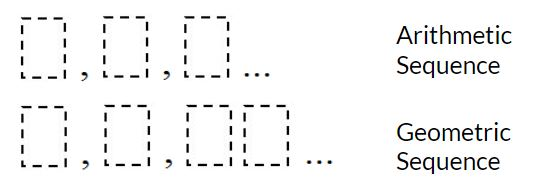# Creating Sequences

Directions: Using the digits 0-9, at most one time each, complete the first three terms of the arithmetic and geometric sequences. What sequences result in the greatest sum of their second terms? (e.g. 3, 5, 7 and 2, 6, 18 would result in a sum of 5 + 6 = 11). What sequences result in the least sum of their second terms?### Hint

The maximum sum would be 17, with the 8 and the 9 digits as the second terms of each sequence. Can this be done? There are only six possible geometric sequences with the given constraint… what are they?

### Answer

The greatest sum is 15. There are three ways to get this: 4, 6, 8 and 3, 9, 27; 5, 7, 9 and 4, 8, 16; and 5, 7, 9 and 1, 8, 64. The least sum is 9. There is one way to get this sum: 1, 3, 5 and 2, 6, 18.

Source: Erick Lee

## What’s Your Sine?

Directions: Use the digits 1 through 9, at most one time each, to fill in …

### 3 comments

1.It seems as though the solution to the least sum is incorrect, as the number 1 is used twice.
I found the patterns 0,3,6 and 1,2,4 with a least sum of 5.

•Here is it true that the geometric sequence would need to be a double digit number?

2.We also agree that the least sum is incorrect, as it violates the rules and uses the 1 twice. We are also debating whether “08” is a two digit number, thus using 1, 3, 5 and 2, 4, 08, and having a sum of 7. Still working! 🙂